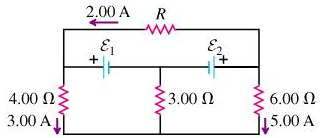# Problem: Find the current in the 3.00 Ω resistor. (Note that three currents are given.)

###### FREE Expert Solution

(1)

Using the Kirchoff's junction rule for the node at the center bottom:

The current through the 3.00 Ω is the sum of the currents through the 4.00 Ω and the 6.00 Ω resistors.

96% (366 ratings)###### Problem DetailsFind the current in the 3.00 Ω resistor. (Note that three currents are given.)